##1. Hyperbola Asymptotics

Look at Hyperbola.html for the definition of the hyperbola and its equation with respect to its principal axes: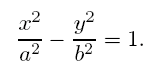For c˛ = a˛+b˛, c = |FF*|/2 being half the focal-distance and e = c/a, which is the eccentricity of the hyperbola (e>1), the angle of its asymptote with the x-axis is given by: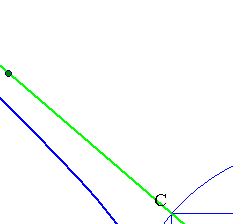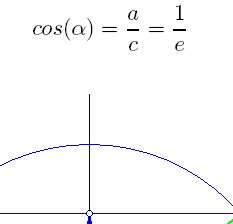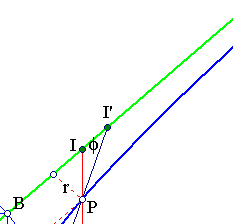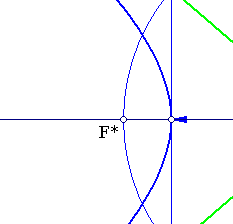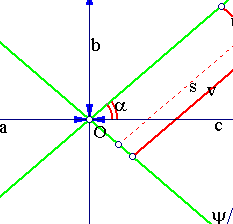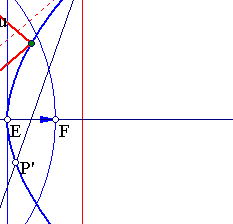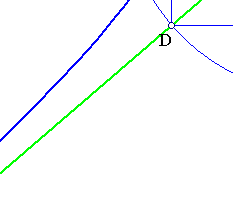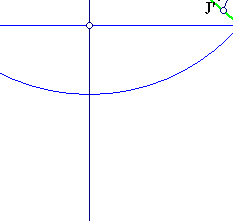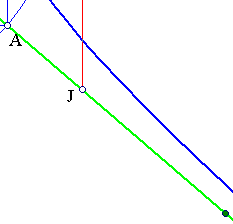Referring the curve to coordinates (u, v), with respect to its asymptotic lines and calculating the relations between the two kinds of coordinates, we find that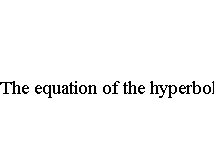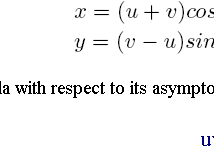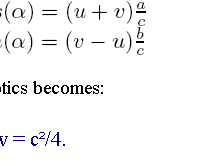The figure shows also the inverse path. How to determine the axes of the hyperbola, knowing its equation uv=c˛/4, with respect to its asymptotic lines: Draw the circle with radius c, find ABCD, F, F* and E. This procedure, of the definition of the hyperbola, from two intersecting lines (playing the role of asymptotics) and positive number c˛ is realized dynamically with EucliDraw in the file HyperbolaWRAsymptotics.html .

##2. A property of asymptotics

For every point P on the hyperbola draw a parallel to the minor axis intersecting the asymptotes at {I,J}. Then
PI*PJ = b2.

Follows from the last basic relation.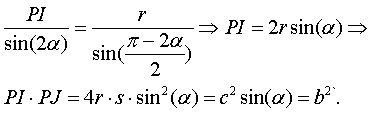Remark This property generalizes in the following way. Take an arbitrary secant through P intersecting the asymptotes at {I',J'} then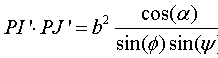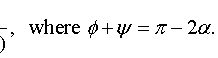In particular, if the secant I'J' moves parallel to itself then the product PI'*PJ' remains constant. Thus, if P comes to the position of P' the product has the same value and this implies that PI'=P'J'.

##3. Construction problem

To construct an hyperbola from its asymptotes and a point P on the curve.

Apply the previous equation to find b. Then construct rectangle ABCD determining the various basic elements (focus etc.) of the hyperbola.